Algebra 1 section of MathBitsNotebook.comPractice Test 2 - CCSS Algebra 1 Interactive Version - Long ResponseDirections:  Questions for Parts II, III, and IV are long response questions. You will need paper, pencil, graph paper or graph grids, and a graphing calculator to work out the problems. Check your answer after solving each problem by clicking the ANSWER button.
Part II: (2 pts)
 25.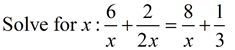26. In the triangle at the right, AB = 3x – 4 and  CD = 4x – 6.  Find, and simplify, an expression for the area of the triangle.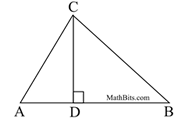27. Given the function c(x) = -| x | - 2, find the maximum value of the c(x).
 28. Using the function g(x) = 2x3 – 10x + 12, find the value of g(-3).
 29. Find the x-intercept(s) of the equation y = 5x2 – 25x.
 30. On a 50 point quiz, the dot plot shows the number of points scored by the students in the class.  Show the relationship between the mean of the scores to the median of the scores.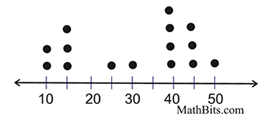31. Combine and simplify: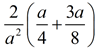32. In a forested area, the number of trees, T, can be expressed as a function of the initial number of trees planted, n, the rate, r, of increase due to natural seeding, and the number of years, x, since the initial planting of the trees.  The function is  T = n(1 + r)x.  If n = 10,000 trees, r = 20% and x = 5 years, how many trees should be growing after 5 years?  (Round to nearest integer.)

Part III:
(4 pts) You need graph paper grids for this section.
 33. The local Tweeter Bird Seed store is running a sale on its most popular bird seed mixture. As you buy additional bags of the seed, you get a reduction in price on the additional bags. •  The first and second bags of seed cost \$20 per bag. •  The third through fifth bags of seed cost \$15 per bag. •  The sixth through tenth bags of seed cost \$10 per bag. •  There is a limit of 10 bags per purchase. a)  Sketch a graph to indicate this sale.  Place the number of bags on the x-axis and the total cost on the y-axis. b)  Is the average rate of change constant across the entire graph?  Explain.
 34. A function is defined as g(x) = -x2 – 2x + 8.  a)  On the grid, sketch a graph labeling any x-intercepts and y-intercepts. b)  State the coordinates of the vertex, and indicate whether the vertex is a maximum or minimum point on the graph.
 35. The Tepid Tea Company is setting up a tea delivery system for on-line customers.  The customer can choose Plan A:  \$12 per carton of tea delivered, or Plan B:  a monthly service fee of \$20, plus \$8.50 per carton of tea delivered. a)  Write a function to model each delivery plan.  (graphing is optional). b)  Describe which plan is a better plan and why.
 36. Graph the inequalities:  2y < 3x + 4  and   y > -x – 1 State one point that satisfies both inequalities.

Part IV:
(6 pts)
 37. Given the function p(x) defined as: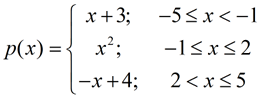a)  Find the value of p(-2). b)  Sketch the graph of p(x). c)  At what x-value in the domain does p(x) have its absolute maximum value?